A train moves from one station to another in 2 hours time. Its speed-time graph during this motion is shown in the figure. The maximum acceleration during the journey is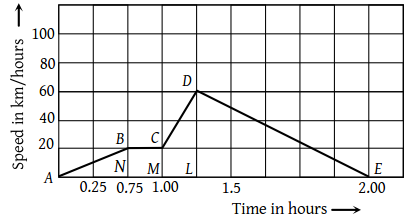(1) 140 km h–2

(2) 160 km h–2

(3) 100 km h–2

(4) 120 km h–2

Concept Questions :-

Graphs
High Yielding Test Series + Question Bank - NEET 2020

Difficulty Level:

A ball is thrown vertically upwards. Which of the following plots represents the speed-time graph of the ball during its height if the air resistance is not ignored

 1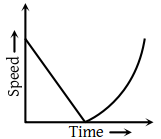2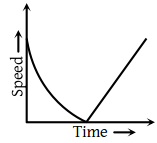3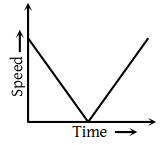4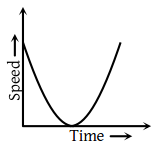Concept Questions :-

Graphs
High Yielding Test Series + Question Bank - NEET 2020

Difficulty Level:

Which graph represents the uniform acceleration

 (1)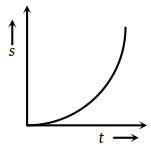(2)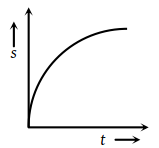(3)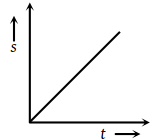(4)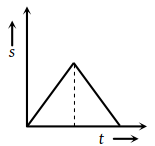Concept Questions :-

Graphs
High Yielding Test Series + Question Bank - NEET 2020

Difficulty Level:

Which of the following velocity-time graphs shows a realistic situation for a body in motion

 1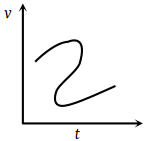2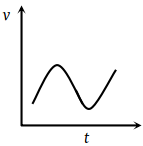3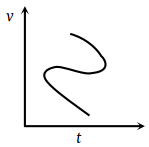4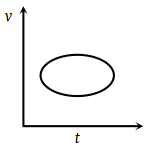Concept Questions :-

Graphs
High Yielding Test Series + Question Bank - NEET 2020

Difficulty Level:

Which of the following velocity-time graphs represent uniform motion

 (1)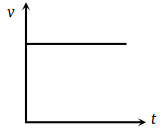(2)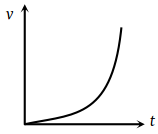(3)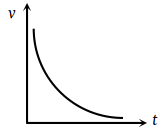(4)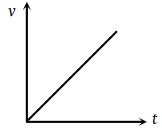Concept Questions :-

Graphs
High Yielding Test Series + Question Bank - NEET 2020

Difficulty Level:

Acceleration-time graph of a body is shown. The corresponding velocity-time graph of the same body is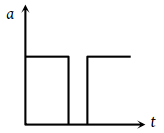(1)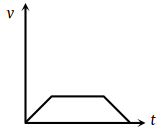(2)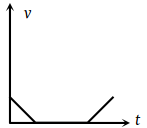(3)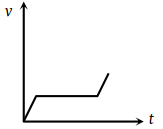(4)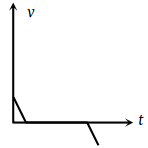Concept Questions :-

Graphs
High Yielding Test Series + Question Bank - NEET 2020

Difficulty Level:

The given graph shows the variation of velocity with displacement. Which one of the graphs given below correctly represents the variation of acceleration with displacement ?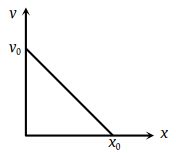1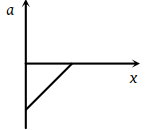2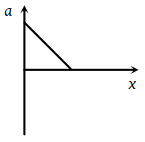3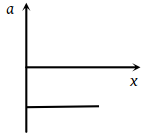4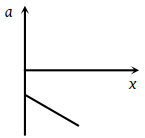Concept Questions :-

Graphs
High Yielding Test Series + Question Bank - NEET 2020

Difficulty Level:

The acceleration-time graph of a body is shown below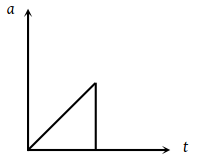The most probable velocity-time graph of the body is

 (1)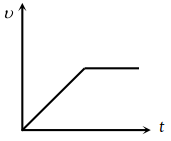(2)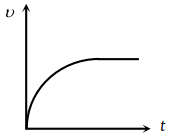(3)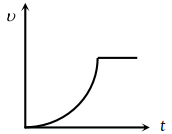(4)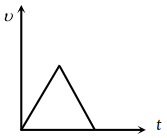Concept Questions :-

Graphs
High Yielding Test Series + Question Bank - NEET 2020

Difficulty Level:

From the following displacement-time graph , find out the velocity of a moving body.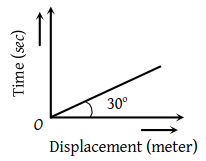1. $\frac{1}{\sqrt{3}}$m/s

2. 3 m/s

3. $\sqrt{3}$m/s

4. $\frac{1}{3}$m/s

Concept Questions :-

Graphs
High Yielding Test Series + Question Bank - NEET 2020

Difficulty Level:

The vt plot of a moving object is shown in the figure. The average velocity of the object during the first 10 seconds is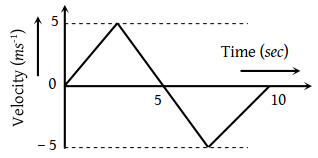(1) 0

(2) 2.5 ms–1

(3) 5 ms–1

(4) 2 ms–1

Concept Questions :-

Graphs